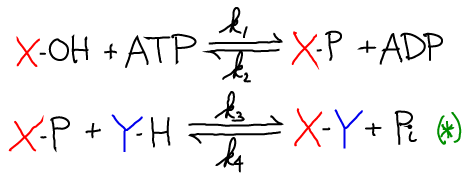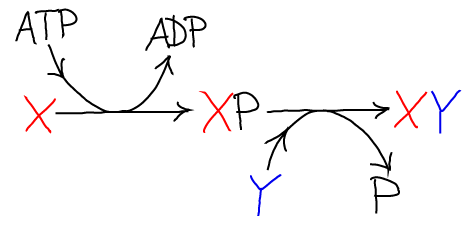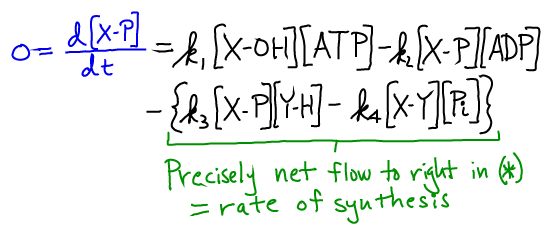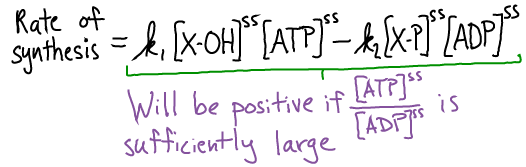# Reaction Coupling

## Biosynthesis is Driven by Free EnergyChemical syntheses in the cell, such as the formation of X-Y above, typically require an input of free energy. Free energy could be supplied directly if there were an over-abundance of reactants (compared to equilibrium) and/or a dearth of products. More commonly, there is a complex sequence of reactions required for synthesis, and free energy drives these intermediate reactions. Below, we see how the hydrolysis of ATP can be coupled to a series of two synthesis reactions. Other carriers could also supply the free energy to drive synthetic reactions.

Note that if a synthetic reaction were favorable (did not require free energy), it would only be necessary for the cell to supply a catalyst.

### Reaction coupling, driven by ATP

An example of the mechanism here is the synthesis of glutamine from glutamic acid, where X-OH = glutmatic acid, Y-H = NH$_3$. Below, -P = -O-PO$_3$.

The synthesis of X-Y can be coupled to ATP hydrolysis by the following two reactionsThe net result of the two reactions is the synthesis of X-Y and the hydrolysis of ATP. Graphically the process looks like this### Steady-state analysis of the coupled reactions

Will the two-step synthesis above really occur under typical cellular conditions, even if the direct synthesis ($X + Y \rightarrow XY$) is unfavorable? We can answer this question using a steady-state analysis of the equations above in a mass action picture. Specifically, we can calculate the rate of formation of XY (and Pi) in the second of the two reactions, which is marked with a green (*). We use a steady-state condition on the intermediate XP:Because the last two terms are precisely the net rate of XY and Pi synthesis - based on Eq (*) - and the four terms together sum to zero in a steady state, we can rewrite the synthesis rate to see the dependence on ATP and ATP:So long as the ratio $\conc{ATP} / \conc{ADP}$ is sufficiently large, the rate of XY synthesis will be positive. And because ATP is an activated carrier that ratio is large.

Bottom line: XY is synthesized at a positive rate if ATP is sufficiently activated.

# Reaction Coupling

## Biosynthesis is Driven by Free EnergyChemical syntheses in the cell, such as the formation of X-Y above, typically require an input of free energy. Free energy could be supplied directly if there were an over-abundance of reactants (compared to equilibrium) and/or a dearth of products. More commonly, there is a complex sequence of reactions required for synthesis, and free energy drives these intermediate reactions. Below, we see how the hydrolysis of ATP can be coupled to a series of two synthesis reactions. Other carriers could also supply the free energy to drive synthetic reactions.

Note that if a synthetic reaction were favorable (did not require free energy), it would only be necessary for the cell to supply a catalyst.

### Reaction coupling, driven by ATP

An example of the mechanism here is the synthesis of glutamine from glutamic acid, where X-OH = glutmatic acid, Y-H = NH$_3$. Below, -P = -O-PO$_3$.

The synthesis of X-Y can be coupled to ATP hydrolysis by the following two reactionsThe net result of the two reactions is the synthesis of X-Y and the hydrolysis of ATP. Graphically the process looks like this### Steady-state analysis of the coupled reactions

Will the two-step synthesis above really occur under typical cellular conditions, even if the direct synthesis ($X + Y \rightarrow XY$) is unfavorable? We can answer this question using a steady-state analysis of the equations above in a mass action picture. Specifically, we can calculate the rate of formation of XY (and Pi) in the second of the two reactions, which is marked with a green (*). We use a steady-state condition on the intermediate XP:Because the last two terms are precisely the net rate of XY and Pi synthesis - based on Eq (*) - and the four terms together sum to zero in a steady state, we can rewrite the synthesis rate to see the dependence on ATP and ATP:So long as the ratio $\conc{ATP} / \conc{ADP}$ is sufficiently large, the rate of XY synthesis will be positive. And because ATP is an activated carrier that ratio is large.

Bottom line: XY is synthesized at a positive rate if ATP is sufficiently activated.